Test: Profit & Loss- 4

# Test: Profit & Loss- 4

Test Description

## 15 Questions MCQ Test Quantitative Aptitude (Quant) | Test: Profit & Loss- 4

Test: Profit & Loss- 4 for Quant 2022 is part of Quantitative Aptitude (Quant) preparation. The Test: Profit & Loss- 4 questions and answers have been prepared according to the Quant exam syllabus.The Test: Profit & Loss- 4 MCQs are made for Quant 2022 Exam. Find important definitions, questions, notes, meanings, examples, exercises, MCQs and online tests for Test: Profit & Loss- 4 below.
Solutions of Test: Profit & Loss- 4 questions in English are available as part of our Quantitative Aptitude (Quant) for Quant & Test: Profit & Loss- 4 solutions in Hindi for Quantitative Aptitude (Quant) course. Download more important topics, notes, lectures and mock test series for Quant Exam by signing up for free. Attempt Test: Profit & Loss- 4 | 15 questions in 30 minutes | Mock test for Quant preparation | Free important questions MCQ to study Quantitative Aptitude (Quant) for Quant Exam | Download free PDF with solutions
 1 Crore+ students have signed up on EduRev. Have you?
Test: Profit & Loss- 4 - Question 1

### CP of 40 articles is equal to the SP of 30 articles. What is the profit/loss percentage?

Detailed Solution for Test: Profit & Loss- 4 - Question 1

CP of 1 article = Re 1

CP of 40 articles = Rs 40

SP of 30 articles = Rs 40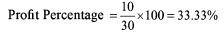Test: Profit & Loss- 4 - Question 2

### Sum of CP’s of two cows is Rs 13,000. Both the cows are sold at a profit of 20% and 40% respectively with their SP’s being the same. What is the difference of CPs of both the cows?

Detailed Solution for Test: Profit & Loss- 4 - Question 2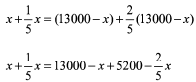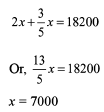Test: Profit & Loss- 4 - Question 3

### A supplier sells 20 pencils at the marked price of 16 pens to a retailer. The retailer, in turn, sells them at the marked price. What is the percentage profit or percentage loss of the retailer?

Detailed Solution for Test: Profit & Loss- 4 - Question 3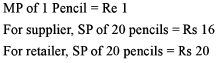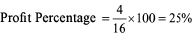Test: Profit & Loss- 4 - Question 4

A shopkeeper sells his goods at its CP only. But he uses 750 grams weight at the place of 1000 gms weight for a kg. What is his net profit percentage?

Detailed Solution for Test: Profit & Loss- 4 - Question 4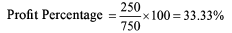Test: Profit & Loss- 4 - Question 5

A wholeseller supplies few chips to a retailer every year. Each chip costs Rs 10,000 to the wholeseller. 5% of the chips are defective and they are to be replaced without charging anything extra. If the wholeseller still makes a profit of 20%, at what price is he selling it to retailer?

Detailed Solution for Test: Profit & Loss- 4 - Question 5

Let No. of chips supplied = 100

For whole seller, Net CP = (10000 x 100 + 5 x 10000) = Rs 1050000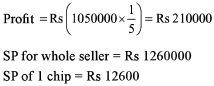Test: Profit & Loss- 4 - Question 6

A shopkeeper wrongly calculates his profit on SP and finds it to be 25%. What is the actual profit percentage?

Detailed Solution for Test: Profit & Loss- 4 - Question 6

Let SP = Rs 100

Profit = Rs 25

CP = Rs 75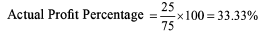Test: Profit & Loss- 4 - Question 7

A shopkeeper sells two cows in such a way that CP of first cow is equal to the SP of second cow and SP of first cow is equal to the CP of second cow. Which of the following is final result after the whole transaction?

Detailed Solution for Test: Profit & Loss- 4 - Question 7

Let CP of 1st Cow = Rs x

CP of 2nd Cow = Rs Y,

Hence net CP = Rs (x + y )

SP of 1st Cow = Rs y

SP of 2nd Cow = Rs X

Net SP = Rs (x + y)

Hence, No Profit No Loss

Test: Profit & Loss- 4 - Question 8

Vinit sells a car to Amit at a profit of 20%. Now Amit sells this car to Vicky at a profit of 12%. And finally Vicky sells this car to Nishu at a loss of 21%. What is the sum of CPs of Amit and Nishu?

Detailed Solution for Test: Profit & Loss- 4 - Question 8

In the whole question, there is nowhere the mention of any Rs figures. Since amount is not given, we cannot calculate any value (in Rs)

Test: Profit & Loss- 4 - Question 9

Amilkman professes to sell milk at its CP only. But still he is making a profit of 20% since he has mixed some amount of water in the milk. What is the percentage of milk in the mixture?

Detailed Solution for Test: Profit & Loss- 4 - Question 9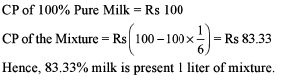Alternatively, if he has initially 10 L of milk, he must have made it 12 L of mixture to get a profit of 20% (since SP per litre = CP per litre). Hence out of 12 litres of mixture, 10 litres is milk and 2 litres is water.

Test: Profit & Loss- 4 - Question 10

While I was shopping in Kamla Nagar market for my baby girl Tatto, I came across two shopkeepers who were giving me a discount of same amount for a top. While first shopkeeper was offering a discount of 14.28%, second shopkeeper was offering a discount of 12.5% over their respective mark-ups. Whose cost price is higher?

Detailed Solution for Test: Profit & Loss- 4 - Question 10

According to the question, 14.28% of Mark up of 1st shopkeeper = 12.5% of mark up of 2nd shopkeeper.
With this we can compare the markup prices.
However nothing can be said about the cost prices of the shopkeepers.

Test: Profit & Loss- 4 - Question 11

A shopkeeper purchases his goods at a discount of 10% on the list price. He normally gives a discount of 6% to his customers. If during a special scheme, he gets an additional discount of 2% on his purchase price, find his approximate profit percentage.

Detailed Solution for Test: Profit & Loss- 4 - Question 11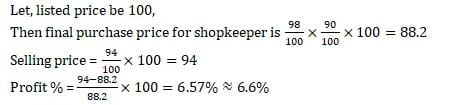Test: Profit & Loss- 4 - Question 12

Anam bought a jute bag at 30% discount on the list price. He then sold it at a price which is 160% of the list price thereby making a profit of Rs 81. What is the list price of the bag?

Detailed Solution for Test: Profit & Loss- 4 - Question 12

Assume that MP = Rs 100

then CP of An am = Rs 70

and his SP = Rs 160

So, his Profit = Rs 90.
Now using unitary method, when profit of Anam = Rs 90, then his MP = 100. So when his MP = Rs 81, his MP = 90.

Test: Profit & Loss- 4 - Question 13

Santa Singh, the local fruit vendor, buys a certain number of oranges at Rs 7 a dozen and equal number at Rs 6 a dozen. He sells the oranges at Rs 7.50 a dozen and makes a profit of Rs 80. How many oranges does he buy?

Detailed Solution for Test: Profit & Loss- 4 - Question 13

C.P. of 2 dozen oranges = Rs. (7 + 6) = Rs. 13.
S.P. of 2 dozen oranges = Rs. 7.5(2)= 15
If profit is Rs. 2,
oranges bought = 2 dozen.
If profit is Rs. 80,
oranges bought = (2/2 x 80) dozens = 80 dozens.

Test: Profit & Loss- 4 - Question 14

Two lots of oranges with equal quantity, one costing Rs 20 per dozen and the other costing Rs 30 per dozen, are mixed together and the whole lot is sold at Rs 24 per dozen. Then what is the profit or loss?

Detailed Solution for Test: Profit & Loss- 4 - Question 14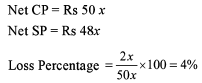Test: Profit & Loss- 4 - Question 15

Navneet is a bananawallah who buys a gross of bananas at Rs 10 a dozen. During transport and storage two dozen bananas get spoilt. If Navneet spends 10% of his outlay on transport costs and wants an overall profit of 20%, at what price must he sell a dozen bananas?

Detailed Solution for Test: Profit & Loss- 4 - Question 15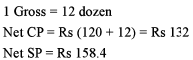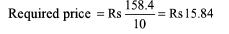## Quantitative Aptitude (Quant)

163 videos|152 docs|131 tests
 Use Code STAYHOME200 and get INR 200 additional OFF Use Coupon Code
Information about Test: Profit & Loss- 4 Page
In this test you can find the Exam questions for Test: Profit & Loss- 4 solved & explained in the simplest way possible. Besides giving Questions and answers for Test: Profit & Loss- 4, EduRev gives you an ample number of Online tests for practice

## Quantitative Aptitude (Quant)

163 videos|152 docs|131 tests